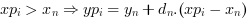Scilab Home page | Wiki | Bug tracker | Forge | Mailing list archives | ATOMS | File exchange
Change language to: English - Português - 日本語 - Русский

Please note that the recommended version of Scilab is 6.0.2. This page might be outdated.
See the recommended documentation of this function

Aide de Scilab >> Interpolation > interp

interp

cubic spline evaluation function

Calling Sequence

[ yp [,yp1 [,yp2 [,yp3]]]] = interp(xp, x, y, d [, out_mode])

Arguments

x,y

real vectors of same size n: Coordinates of data points on which the interpolation and the related cubic spline (called s(X) in the following) or sub-spline function is based and built.

d

real vector of size(x): The derivative s'(x). Most often, s'(x) will be priorly estimated through the function splin(x, y,..)

out_mode

(optional) string defining s(X) for X outside. Possible values: "by_zero" | "by_nan" | "C0" | "natural" | "linear" | "periodic"

xp

real vector or matrix: abscissae at which Y is unknown and must be estimated with s(xp)

yp

vector or matrix of size(xp): yp(i) = s(xp(i)) or yp(i,j) = s(xp(i,j))

yp1, yp2, yp3

vectors (or matrices) of size(x): elementwise evaluation of the derivatives s'(xp), s''(xp) and s'''(xp).

Description

The cubic spline function s(X) interpolating the (x,y) set of given points is a continuous and derivable piece-wise function defined over. It consists of a set of cubic polynomials, each onebeing defined onand connected in values and slopes to both its neighbours. Thus, we can state that for each, such that. Then, interp() evaluates s(X) (and s'(X), s''(X), s'''(X) if needed) at xp(i), such thatThe out_mode parameter set the evaluation rule for extrapolation, i.e. for xp(i) outside:

"by_zero"

an extrapolation by zero is done

"by_nan"

extrapolation by Nan (%nan)

"C0"

the extrapolation is defined as follows :"natural"

the extrapolation is defined as follows (being the polynomial defining s(X) on)"linear"

the extrapolation is defined as follows :"periodic"

s(X) is extended by periodicity:Examples

// see the examples of splin and lsq_splin

// an example showing C2 and C1 continuity of spline and subspline
a = -8; b = 8;
x = linspace(a,b,20)';
y = sinc(x);
dk = splin(x,y);  // not_a_knot
df = splin(x,y, "fast");
xx = linspace(a,b,800)';
[yyk, yy1k, yy2k] = interp(xx, x, y, dk);
[yyf, yy1f, yy2f] = interp(xx, x, y, df);
clf()
subplot(3,1,1)
plot2d(xx, [yyk yyf])
plot2d(x, y, style=-9)
legends(["not_a_knot spline","fast sub-spline","interpolation points"],...
[1 2 -9], "ur",%f)
xtitle("spline interpolation")
subplot(3,1,2)
plot2d(xx, [yy1k yy1f])
legends(["not_a_knot spline","fast sub-spline"], [1 2], "ur",%f)
xtitle("spline interpolation (derivatives)")
subplot(3,1,3)
plot2d(xx, [yy2k yy2f])
legends(["not_a_knot spline","fast sub-spline"], [1 2], "lr",%f)
xtitle("spline interpolation (second derivatives)")// here is an example showing the different extrapolation possibilities
x = linspace(0,1,11)';
y = cosh(x-0.5);
d = splin(x,y);
xx = linspace(-0.5,1.5,401)';
yy0 = interp(xx,x,y,d,"C0");
yy1 = interp(xx,x,y,d,"linear");
yy2 = interp(xx,x,y,d,"natural");
yy3 = interp(xx,x,y,d,"periodic");
clf()
plot2d(xx,[yy0 yy1 yy2 yy3],style=2:5,frameflag=2,leg="C0@linear@natural@periodic")
xtitle(" different way to evaluate a spline outside its domain")• splin — cubic spline interpolation
• lsq_splin — weighted least squares cubic spline fitting

History

 Version Description 5.4.0 previously, imaginary part of input arguments were implicitly ignored.